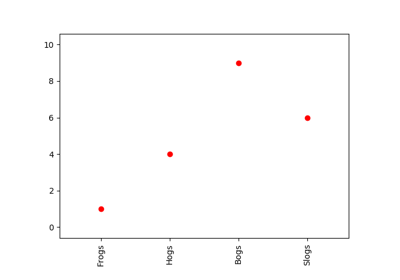# matplotlib.pyplot.margins¶

`matplotlib.pyplot.``margins`(*args, **kw)[source]

Set or retrieve autoscaling margins.

signatures:

```margins()
```

returns xmargin, ymargin

```margins(margin)

margins(xmargin, ymargin)

margins(x=xmargin, y=ymargin)

margins(..., tight=False)
```

All three forms above set the xmargin and ymargin parameters. All keyword parameters are optional. A single argument specifies both xmargin and ymargin. The padding added to the end of each interval is margin times the data interval. The margin must be a float in the range [0, 1].

The tight parameter is passed to `autoscale_view()` , which is executed after a margin is changed; the default here is True, on the assumption that when margins are specified, no additional padding to match tick marks is usually desired. Setting tight to None will preserve the previous setting.

Specifying any margin changes only the autoscaling; for example, if xmargin is not None, then xmargin times the X data interval will be added to each end of that interval before it is used in autoscaling.

## Examples using `matplotlib.pyplot.margins`¶Rotating custom tick labels